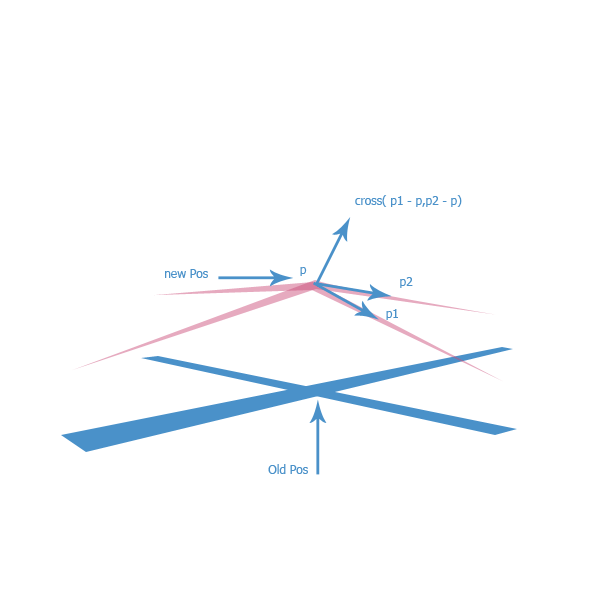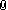## Recommended Posts

Hi, guru's!

I need advice or dig direction.

Problem:

Make shader material (glsl) and in vertex shader i change vertexes position. It work ok and results is pretty.

BUT (yes, again this damn "BUT")In fragment shader i need normal for compute reflection, diffuse and etc.

Source mesh is flat, and all normal look up and equal.  Vertex shader change vertex position, but normals stay not changed.

How i can recalculate (or get somewhere) ) new normals?  In vertex shader i have only ONE current vertex, but for calculate normal we need face (i thinki.e. 3 vertexes).

How get new normal in current vertex in new position? Help please!

p. s.

http://www.babylonjs.com/cyos/  Template "wave"  we have near situation, but normal (as i understand) not recalc and in distortion result we not get real light piscture.... or have?  Trap...

##### Share on other sites

p. s.

http://www.babylonjs.com/cyos/  Template "wave"  we have near situation, but normal (as i understand) not recalc and in distortion result we not get real light piscture.... or have?  Trap...

OK, so I don't know what your shaders look like, but let's use the example of the wave effect you link to. In that case you could easily rotate the normal without looking at adjacent vertices - you have a function used to offset a vertex, so the normal that is used as in input to the vertex shader needs to be rotated by the normal to the offset function (the amount of rotation needs to be scaled by the amount of that offset nb: the scaling of the offset may not be linear... I'm too lazy to do the maths).

##### Share on other sites

http://www.babylonjs.com/cyos/#1YDNAM#5

it is a simple way for find  a new normal after change pos

step 1 : you need find your function like

new_pos = function(old_pos);

step 2 : call function for 2 new pos

new_pos1 = function(old_pos+vec3(0.,0.,x));

new_pos2 = function(old_pos+vec3(x,0.,0.0));

x: 0.2 : need some test for diffrent object:)

step 3 :

vec3 nrm = -1.0*normalize( cross(new_pos1 - new_pos ,new_pos2 - new_pos));

vNormal = nrm*0.5+normal*0.5;

http://www.babylonjs.com/cyos/#1YDNAM#6

##### Share on other sites

http://www.babylonjs.com/cyos/#1YDNAM#5

it is a simple way for find  a new normal after change pos

step 1 : you need find your function like

new_pos = function(old_pos);

step 2 : call function for 2 new pos

new_pos1 = function(old_pos+vec3(0.,0.,x));

new_pos2 = function(old_pos+vec3(x,0.,0.0));

x: 0.2 : need some test for diffrent object:)

step 3 :

vec3 nrm = -1.0*normalize( cross(new_pos1 - new_pos ,new_pos2 - new_pos));

vNormal = nrm*0.5+normal*0.5;

http://www.babylonjs.com/cyos/#1YDNAM#6

Thank you!!!  I need think about it and make testsNow i wonder can i make function x2=f(x1), because we dont know how is vertexes sequences in shader.   Look, we have quad surface (2 triangles). Sequence may be p1-p2-p3,p1-p3-p4  or p1-p2-p4,p2-p3-p4 or another variants.

And x2 (in midn shader) and x2 (in us mind) may be different. Or i mistaken?

##### Share on other sitesmaybe :

cross(p1-p,p2-p)*0.25+cross(p2-p,p3-p)*0.25+cross(p3-p,p4-p)*0.25+cross(p4-p,p1-p)*0.25

##### Share on other sitestest1.gif

maybe :

cross(p1-p,p2-p)*0.25+cross(p2-p,p3-p)*0.25+cross(p3-p,p4-p)*0.25+cross(p4-p,p1-p)*0.25

O! Thank you for picture and help!

I understand vector of you mind, but how i get p2 or p1, if can write y=f(x). How vertex get Shader in next iteration?##### Share on other sites

just move the current position to the sides like vec3(0.,0.,0.1) or vec3(0.1,0.,0.) maybe you change that pos per case but this way is not final answer just closer to the final normal

** you can find  flat normal with normalize(cross(dFdx(pos*-1.),dFdy(pos)))

##### Share on other sites

just move the current position to the sides like vec3(0.,0.,0.1) or vec3(0.1,0.,0.) maybe you change that pos per case but this way is not final answer just closer to the final normal

** you can find  flat normal with normalize(cross(dFdx(pos*-1.),dFdy(pos)))

Thank you again!

While i can't get good result (small shiffts i was try). Will continue dig and tell here about progress.... or lack of progress##### Share on other sites

NasimiAsl, hi!

I made thisYou method is right.

`float	delta		=	0.08;vec3    p		=	position;vec3 	p1		=	normalize(vec3(p.x-delta,	yFXYZ(vec3(p.x-delta,p.yz)),	p.z));vec3 	p2		=	normalize(vec3(p.x,		yFXYZ(vec3(p.xy,p.z-delta)),	p.z-delta));	vNormal			=	normalize(cross((p1-p),(p2-p)));`

Thank's!

But i have other absorbing problem - near zero point of mesh we have "quirk edge" effect. I think because, when number's is very small we have math mistakes in GPU.

I cant beat this (three day's test for catch problem). And invented only shift all mesh away from zero pointYes, shame with me for this "solution", but while this work))

## Join the conversation

You can post now and register later. If you have an account, sign in now to post with your account.
Note: Your post will require moderator approval before it will be visible.×   Pasted as rich text.   Paste as plain text instead

Only 75 emoji are allowed.